Related Articles
Logistic Regression in R Programming
• Last Updated : 05 Jun, 2020

Logistic regression in R Programming is a classification algorithm used to find the probability of event success and event failure. Logistic regression is used when the dependent variable is binary(0/1, True/False, Yes/No) in nature. Logit function is used as a link function in a binomial distribution.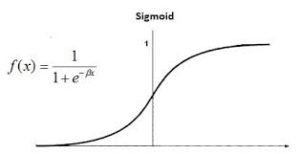Logistic regression is also known as Binomial logistics regression. It is based on sigmoid function where output is probability and input can be from -infinity to +infinity.

#### Theory

Logistics regression is also known as generalized linear model. As it is used as a classification technique to predict a qualitative response, Value of y ranges from 0 to 1 and can be represented by following equation: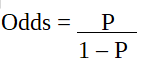`p` is probability of characteristic of interest. The odds ratio is defined as the probability of success in comparison to the probability of failure. It is a key representation of logistic regression coefficients and can take values between 0 and infinity. Odds ratio of 1 is when the probability of success is equal to the probability of failure. Odds ratio of 2 is when the probability of success is twice the probability of failure. Odds ratio of 0.5 is when the probability of failure is twice the probability of success.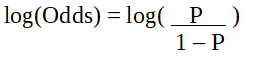Since we are working with a binomial distribution(dependent variable), we need to choose a link function that is best suited for this distribution.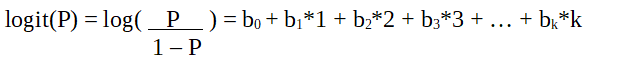It is logit function. In the equation above, the parenthesis is chosen to maximize the likelihood of observing the sample values rather than minimizing the sum of squared errors(like ordinary regression). The logit is also known as a log of odds. The logit function must be linearly related to the independent variables. This is from equation A, where the left-hand side is a linear combination of x. This is similar to the OLS assumption that y be linearly related to x.

Variables b0, b1, b2 … etc are unknown and must be estimated on available training data. In a logistic regression model, multiplying b1 by one unit changes the logit by b0. The P changes due to a one-unit change will depend upon the value multiplied. If b1 is positive then P will increase and if b1 is negative then P will decrease.

#### The Dataset

`mtcars`(motor trend car road test) comprises fuel consumption, performance and 10 aspects of automobile design for 32 automobiles. It comes pre installed with `dplyr` package in R.

 `# Installing the package``install.packages(``"dplyr"``)``  ` `# Loading package``library(dplyr)``  ` `# Summary of dataset in package``summary(mtcars)`

#### Performing Logistic regression on dataset

Logistic regression is implemented in R using `glm()` by training the model using features or variables in the dataset.

 `# Installing the package``install.packages(``"caTools"``)    ``# For Logistic regression``install.packages(``"ROCR"``)       ``# For ROC curve to evaluate model``   ` `# Loading package``library(caTools)``library(ROCR) ``  ` `# Splitting dataset``split <``-` `sample.split(mtcars, SplitRatio ``=` `0.8``)``split``  ` `train_reg <``-` `subset(mtcars, split ``=``=` `"TRUE"``)``test_reg <``-` `subset(mtcars, split ``=``=` `"FALSE"``)``  ` `# Training model``logistic_model <``-` `glm(vs ~ wt ``+` `disp, ``                      ``data ``=` `train_reg, ``                      ``family ``=` `"binomial"``)``logistic_model``  ` `# Summary``summary(logistic_model)``  ` `# Predict test data based on model``predict_reg <``-` `predict(logistic_model, ``                       ``test_reg, ``type` `=` `"response"``)``predict_reg  ``  ` `# Changing probabilities``predict_reg <``-` `ifelse(predict_reg >``0.5``, ``1``, ``0``)``  ` `# Evaluating model accuracy``# using confusion matrix``table(test_reg\$vs, predict_reg)``  ` `missing_classerr <``-` `mean(predict_reg !``=` `test_reg\$vs)``print``(paste(``'Accuracy ='``, ``1` `-` `missing_classerr))``  ` `# ROC-AUC Curve``ROCPred <``-` `prediction(predict_reg, test_reg\$vs) ``ROCPer <``-` `performance(ROCPred, measure ``=` `"tpr"``, ``                             ``x.measure ``=` `"fpr"``)``  ` `auc <``-` `performance(ROCPred, measure ``=` `"auc"``)``auc <``-` `auc@y.values[[``1``]]``auc``  ` `# Plotting curve``plot(ROCPer)``plot(ROCPer, colorize ``=` `TRUE, ``     ``print``.cutoffs.at ``=` `seq(``0.1``, by ``=` `0.1``), ``     ``main ``=` `"ROC CURVE"``)``abline(a ``=` `0``, b ``=` `1``)``  ` `auc <``-` `round``(auc, ``4``)``legend(.``6``, .``4``, auc, title ``=` `"AUC"``, cex ``=` `1``)`

wt influences dependent variables positively and one unit increase in wt increases the log of odds for vs =1 by 1.44. disp influences dependent variables negatively and one unit increase in disp decreases the log of odds for vs =1 by 0.0344. Null deviance is 31.755(fit dependent variable with intercept) and Residual deviance is 14.457(fit dependent variable with all independent variable). AIC(Alkaline Information criteria) value is 20.457 i.e the lesser the better for the model. Accuracy comes out to be 0.75 i.e 75%.

Model is evaluated using the Confusion matrix, AUC(Area under the curve), and ROC(Receiver operating characteristics) curve. In the confusion matrix, we should not always look for accuracy but also for sensitivity and specificity. ROC and AUC curve is plotted.

Output:

• Evaluating model accuracy using confusion matrix: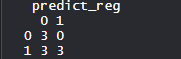There are 0 Type 2 errors i.e Fail to reject it when it is false. Also, there are 3 Type 1 errors i.e rejecting it when it is true.

• ROC curve: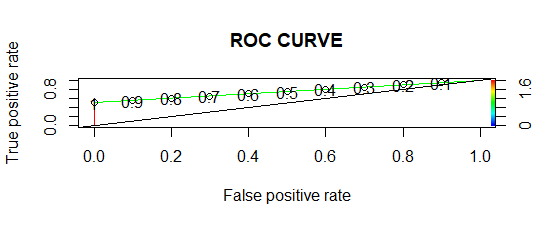In ROC curve, the more the area under the curve, the better the model.

• ROC-AUC Curve: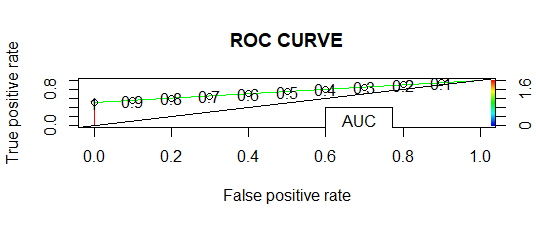AUC is 0.7333, so the more AUC is, the better the model performs.My Personal Notes arrow_drop_up